Our current goal is to gain skill at modeling 2-dimensional motion, especially projectiles. (Note: The motion of all projectiles is two dimensional (2D), as is the motion of many other things, like billiard balls. The path of any baseball or bullet is parabolic and thus two dimensional, given that we treat is as a standard projectile. A projectile, you will recall, is an object upon which a single force acts, with that force being gravity. That means that if a strong wind blows a bullet, while in flight, the bullet is no longer considered a projectile (the wind is another force) and we lose the ability to model it in two dimensions.)

Imagine that someone kicks a football from the ground, at some angle, and it lands downfield. A video of the kick is analyzed, and the 2-dimensional position of the ball is plotted on a graph, with data points 1/24th of a second apart. (Why 1/24th of a second? Because this is the frame rate of the video camera.) The data is then plotted to show X vs t (horizontal position versus time) and Y vs t (vertical position versus time), with distances in meters and time in seconds. These two graphs (or scatter plots) yield the following two equations:

X vs t : y = 16.853x

Y vs t : y = -4.9x2 + 14.141x

which you promptly interpret as:

X vs t : ∆x = 16.853t, which is a simplified form of the standard model ∆x = vit + ½ at2  where vi = 16.853 m/s in the horizontal direction (more specifically, forward) and a = 0 m/sin the horizontal direction.

Y vs t : ∆y = 14.141t - 4.9t2, which is a form of the same standard model, where vi = 14.141 m/s in the vertical direction (more specifically, upward) and a = -9.8 m/s2 in the vertical direction (more specifically, downward).

Based on these two models (or should we call them two parts of a single model?), we hope to verify how long the ball was in flight, i.e. the time at which it landed.

The ∆y model seems better suited to the task, as we know the total change in height (∆y) for a ball that lands at its initial height is simply zero. Thus, we can write, 0 = 14.141t - 4.9t2, where it is understood that the t now represents the time at which ∆y = 0. Using a bit of algebra, we re-arrange this equation to read 4.9t = 14.141, thus t = 2.886 seconds. Sure enough, this coincides with the flight time from the video camera's clock. (Actually, the clock read 2:35.05 when the ball left the ground and 2:37.94 when it landed, which corresponds to a flight time of 2.89 seconds. It's the same time, rounded.)

It is now trivial to work out the range of the ball.

We can easily use the model ∆x = 16.853t to find the horizontal displacement for any time between our starting and stopping times. For a time of 2.886 seconds, ∆x = 16.853(2.886) = 48.638 meters. This is our range.

Quick question. How far forward had the ball moved after 1.000 second? Yep, 16.853 meters.

And how far upward? 9.241 meters. (Can you see where that came from?)

Okay, now let's use vectors to work out the ball's initial velocity, both magnitude and direction.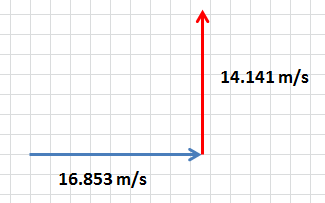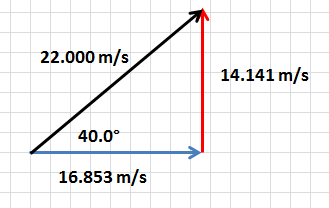On the left, we have our two initial velocities represented as vectors. We call these the horizontal and vertical components of the (true) velocity.

On the right, our two vector components have been added, resulting in the our velocity (the black vector). The Pythagorean theorem gives us its length, 22.000 meters, and trigonometry [tan-1 (14.141/16.853)] gives us our direction, 40.0° above the horizon. We're done.

Can we use vectors with displacement, too? So far, we've used them only to help us model the velocity.

The answer is yes, but they are less useful.

I could ask you, what is the ball's displacement at t = 1.25 second?

Well ... try to work this out before reading onward. Use vectors.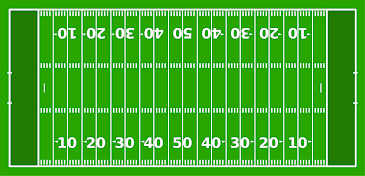First, we use our models to obtain the horizontal and vertical components of the displacement, at t = 1.25 s.

∆x = 16.853(1.25) = 21.07 meters

∆y = 14.141(1.25) - 4.9(1.252) = 10.02 meters

Now we add these two vectors. You probably realize that drawing the vectors is not necessary. If you'd like, you can simply imagine connecting them head-to-tail and drawing in the resultant vector (the hypotenuse, if you'd like). But I'll draw them out anyway. It's good practice.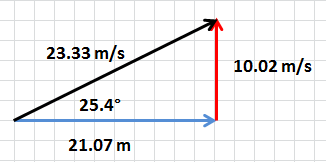We have our answer, and you might be thinking, "The vectors seemed rather useful in finding the overall displacement here." You're right. They were. I say they're not too useful with displacement because you're rarely asked to find the displacement of a projectile for any time other than the landing time. And to do that, you don't need to add vectors. Look back to the example we did earlier on this page, if you need to.

Geometrical representations of vectors... Remember, they are a tool. A means toward an end. We use them when we need to. If you don't need them to model something or work something out, great. If you do, then use them.

Okay, so the analysis above was based on data, collected, plotted and turned into horizontal and vertical models of motion. What we'll look at next is what you're more-likely to find in a standard physics textbook.

Imagine that a football is kicked from the ground, giving it an initial velocity of 28.0 m/s at an angle of 32° above the horizon. Find the ball's range.

Here, we begin not with the horizontal and vertical components of the velocity but rather the overall velocity. We must work out the components, because they are what we use in our models. It's a simple task, involving trigonometry. Let's do it.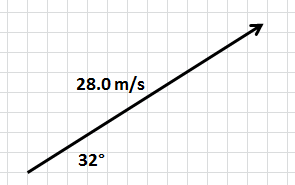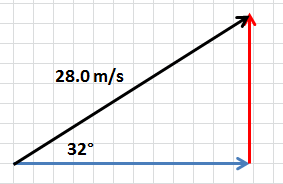On the left, we have our velocity vector.

On the right, I've sketched in the horizontal and vertical components of the velocity vector. You might also say that I "completed the triangle". It helps if the vectors are drawn on a grid or graph paper.

To find the blue horizontal component of the velocity (vx), we use the cosine function.

We find that vx = 23.7 m/s.

To find the red vertical component of the velocity (vy), we use the sine function.

We find that vy = 14.8 m/s.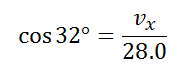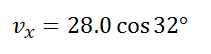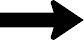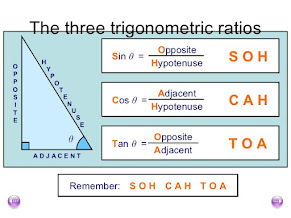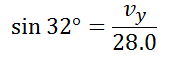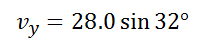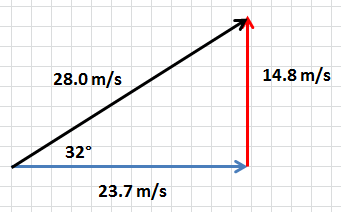X vs t : ∆x = 23.7t, a simplified form of ∆x = vit + ½ at2

Y vs t : ∆y = 14.8t - 4.9t2, a form of the same standard model, where we assume that, because we're on Earth, the local acceleration of gravity is -9.8 m/s/s. (So, you'll always use -4.9 in the model unless we're modeling something that's not on Earth.)

Once you work through several examples of this type of problem, you get to be very fast at generating the two models (horizontal and vertical). Personally, in my calculator, I'll quickly multiply the velocity ("hypotenuse") by sine and cosine of the given angle to get its two components. Then I'll directly write down my two models.

We're not done with this problem. The goal was to find the projectile's range. As before, the best method is to use the y model to find the time associated with a y of 0, which implies the ball has returned to the ground. Here, we solve 0 = 14.8t - 4.9t2 to find that t = 3.02 seconds. Then we can use the ∆x model, evaluated at that time, to find that the range ∆x = 71.6 m.

Hey, can you find the time at which the football reaches its greatest height? And can you find the maximum height itself? Use the various models you know. There's more than one way to find these values, just so you know.

Click on the image for the answers, after attempting it yourself first.

Alright, let's work through another standard textbook problem.

A small metal ball rolls off a 1.2-m high table going 6.5 m/s. How long does it take the ball to strike the floor, and what is its range?

It was unnecessary to state an angle in the problem, because the ball leaves the table horizontally. (Technically, this is an angle of 0°.) Our initial velocity is our horizontal velocity component. (Re-read the last sentence.) There is no vertical velocity component, initially. Only after it begins falling does the ball acquire a velocity in the vertical direction.

The vector representation is trivial.

X vs t : ∆x = 6.5t, a simplified form of ∆x = vit + ½ at2, where a = 0 in the horizontal direction

Y vs t : ∆y = - 4.9t2, a form of the same standard model, where vi = 0 and, as always (on Earth), a = -9.8 m/s/s.

We can find the time it takes for the ball to strike the ground using the ∆y model. The student that doesn't think very carefully about what things mean might set ∆y = 0. That would be a mistake. When the ball lands, how much has its height changed, overall? It's dropped 1.2 meters. So, ∆y = -1.2 m.

So, at what time has the ball dropped 1.2 m? The model, -1.2 = -4.9t2, can be solved to yield t = 0.495 second.

The range is simply ∆x = 6.5(0.495) = 3.22 meters.

So, the ball takes 0.495 seconds to fall 1.2 meters. How long does it take to fall half of this distance?

Is it half of 0.495 seconds? Is the relationship between vertical displacement and time linear? Think about it, then work it out.

Okay, and now, just for practice, let's draw the vector components of the velocity, at t = 0.15 seconds.

Well, the horizontal component is easy. It's what we started with. The horizontal velocity of a projectile never changes. But how long should our vertical component be? Can you work this out? Try it before reading on.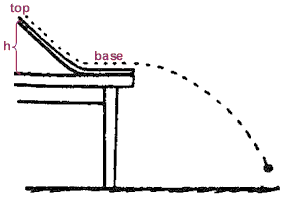Ignore the ramp. All we care about is that the ball is going 6.5 m/s when it leaves the table.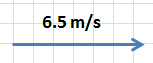This is a salt flat in Bolivia. It's pretty (reflective).

To find the vertical component of the velocity (or, as an abbreviation, the "vertical velocity"), we can use the model vf = at + vi. As always (on Earth), a = -9.8 m/s/s for projectiles. And, for this particular problem, vi = 0 in the vertical direction. So, to find the vertical velocity at some time, start with 0 m/s and subtract 9.8 for every second of falling. For this problem, vf = (-9.8)(0.15) + 0 = -1.47 m/s.

At t = 0.15 s, we have:

These are easy enough to add.

And the direction of our velocity vector -- the direction the ball is actually moving at t = 0.15 s -- can be found using tan-1(1.47/6.5) = 12.7° below the horizon.

Now, you find the velocity of the ball at the moment it reaches the ground. Note: When people ask you to do this, they do NOT want you to answer "0 m/s". Once it strikes the ground, admittedly it does come to rest, but that's obvious. Find the maximum velocity reached just before it begins to slow upon touching the ground. This is done by finding the velocity at the landing time, here t = 0.495 second.

Δx = vavgt               Δx = vit + ½ at2

vf = at + vi               vf2 = vi2 + 2aΔx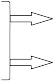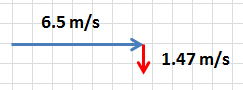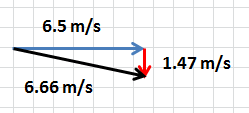Stop for a moment to consider what you've accomplished so far, going back to the first webpage you read on this site.

My goal was not to give you four equations, tell you to memorize them, and then show you how to solve textbook problems with them. This approach (believe me) leads to shallow understanding and frustration.

My goal went deeper. I wanted to help you develop a way of thinking about motion and our attempts at modeling it. The equations are not just equations. They are models. They make statements about the world.

And, for a moment, appreciate the fact that over all these pages of content, we've only really worked with four equations (models). And those four originated with just two. Really, all of the modeling we've done is based upon two equations! And those two equations are really no more than definitions!

Of course, in addition to our mathematical models, I tried to show you how geometrical representations of  vectors (i.e. actually drawing vectors) can be useful in representing certain aspects of motion. And they can help us work out what to substitute into our mathematical models.

Now, should you, at this point, be a master of modeling one- and two-dimensional motion? No, certainly not. If you are, you're more clever than I am. But you should be significantly better than before you began studying physics. Just like any sport, you get better over time, with practice. Keep growing!

Now ... onward!

Δx = vavgt               Δx = vit + ½ at2

vf = at + vi               vf2 = vi2 + 2aΔxClick on the link above for an excellent tool to help you visualize and understand vector components.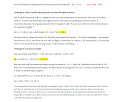Let's work through a couple more problems together. Then I'll ask you to practice on your own.

A ball is launched from the ground at 35.0 m/s at 20.0° above the horizon. Find the ball's range, and find its velocity at 0.85 seconds.

We want to find ∆x at the time of landing, and we want to find v at t = 0.85 s.

It's a projectile, so we'll model the horizontal and vertical motions separately. This requires "resolving" the velocity into its horizontal and vertical components.

horizontal vi = 35.0 cos(20.0°) = 32.889 m/s

vertical vi = 35.0 sin(20.0°) = 11.971 m/s

Of our 4 kinematic equations, ∆x = vit  + ½at2 proves the most useful for finding ∆x.

Our horizontal and vertical models become:

∆x = 32.889t

∆y = 11.971t + ½(-9.8)t2 = 11.971t - 4.9t2

The ball has landed when ∆y = 0. The associated time can be worked out from 0 = 11.971t - 4.9t2.

We find that t = 2.443 seconds.

At this time, ∆x = 32.889(2.443) = 80.35 meters. That's the ball's range: 80.35 meters forward.

To find its' velocity, we'll use the model vf = at + vi.

The horizontal velocity is constant; there is no acceleration horizontally. At t = 0.85 s and every other time, vx = 32.889 m/s. The vertical velocity, on the other hand, is changing. At t = 0.85 s, it happens to be:

vy = (-9.8)0.85 + 11.971 = 3.641 m/s

Okay, so at t = 0.85 s, the ball is moving 32.889 m/s forward and 3.641 m/s upward. Well, that's a useful way to think of its motion. The actual velocity is the vector sum of those two components. Earlier, we took the velocity and "broke" it into components. We'll now put the components back together to get the velocity, with its proper direction.

The Pythagorean theorem leads to a velocity of 33.090 m/s, with direction tan-1(3.641/32.889) = 6.32° above the horizon. Our answer: At t = 0.85 s, the ball's velocity is 33.09 m/s at 6.32° above the horizon.

A ball thrown horizontally at 22.2 m/s from the roof of a building lands 36.0 m from the base of the building. How high is the building?

While this problem is structured differently than what we're used to (we're not being asked to find the ball's range), we ARE still modeling a projectile. To track its position with time, we'll still use:

∆x = vit + ½at2

But let's not rush ahead. Let's use the problem-solving guidelines we've discussed in the past. They remind us to visualize the problem first. Try to understand what is happening and what we're looking for. That's pretty straightforward here, I think. We're trying to find ∆y; the absolute value of ∆y will be the building's height.

(I didn't follow the problem-solving guidelines with the previous practice problem, did I? But I want to now. Realize that the steps are simply suggestions to assist you. If they slow you down but don't affect your accuracy, then you may not need to follow them closely. If you come across a physics problem that seems unfamiliar or confusing, then it might benefit you to use the guidelines. That is, use them when they help. Do you think a professional engineer writes down every step of a problem he or she is solving? No no. The better at problem-solving you become, the more you do "in your head" and the more shortcuts you take. Understood?)

We'll now identify, write down and label the various quantities we're working with.

horizontal vi = 22.2 m/s; ∆x = 36.0 m; vertical a = -9.8 m/s2

The next step is to choose a model. We've already identified ∆x = vit + ½at2 as a good one.  (It wouldn't be our choice for modeling the projectile's velocity with time, but it is appropriate for modeling displacement with time.) Modeling the horizontal and vertical motions separately, we can write:

∆x = 22.2t

∆y = -4.9t2

(If the ball was launched at an angle, this is the point at which we'd use sine and cosine to find the initial velocity's components. The components are what belong in the models.)

Now, I've selectively input vi and a into our models so they'd look familiar. I'll now substitute in the one other quantity we know, ∆x.  For any value we substitute into ∆x, we'll be able to calculate the corresponding time at which it has that particular horizontal displacement.

36 = 22.2t

∆y = -4.9t2

The horizontal equation can be solved to obtain t = 1.62 s. This is how long the ball takes to move forward 36.0 m and is also the time the ball is air born.

We can now use the ∆y model to find the vertical displacement in that period of time.

∆y = -4.9(1.622) = -12.86 meters.

If the ball fell 12.86 meters, then the building must have been 12.86 meters high.

It's a bit messy to have two qualifiers attached to a quantity. For example, the velocity 22.2 m/s is both horizontal and initial. While I wrote "horizontal vi", many people simply use two subscripts, writing instead vxi.

If you're using i and j vector notation to indicate direction, you avoid the problem. In this case, we'd write vi = 22.2 i (m/s). Likewise, for acceleration, we'd write a = -9.8 j (m/s2).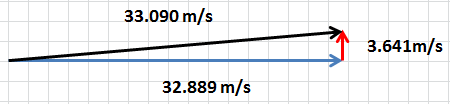practice   problems

problems

Forces

Kinematics